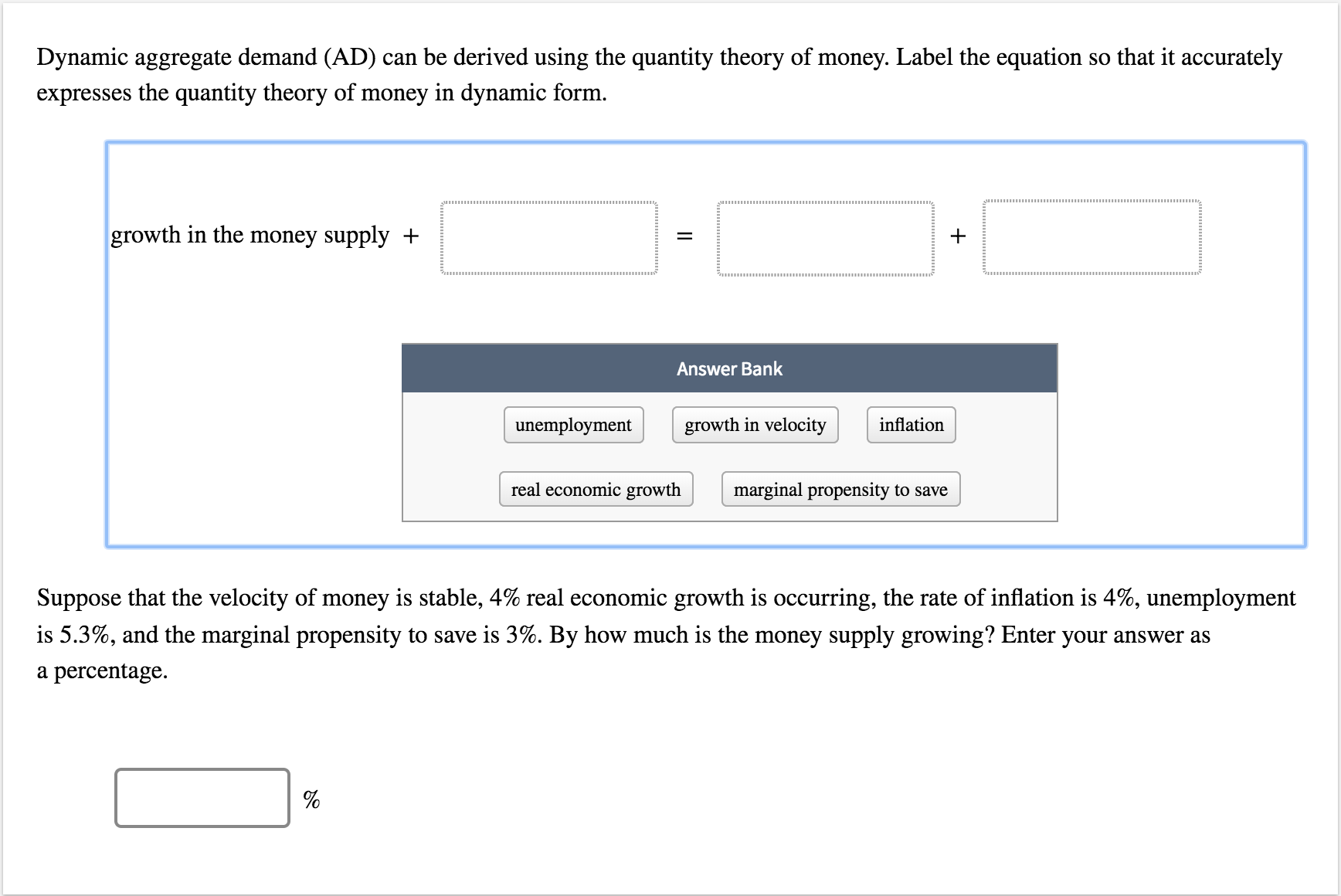# Dynamic aggregate demand (AD) can be derived using the quantity theory of money. Label the equation so that it accurately expresses the quantity theory of money in dynamic form growth in the money supply -+ Answer Bank unemployment growth in velocity inflation real economic growth marginal propensity to save Suppose that the velocity of money is stable, 4% real economic growth is occurring, the rate of inflation is 4%, unemployment is 5.3%, and the marginal propensity to save is 3%. By how much is the money supply growing? Enter your answer as a percentage.

Questionhelp_outlineImage TranscriptioncloseDynamic aggregate demand (AD) can be derived using the quantity theory of money. Label the equation so that it accurately expresses the quantity theory of money in dynamic form growth in the money supply -+ Answer Bank unemployment growth in velocity inflation real economic growth marginal propensity to save Suppose that the velocity of money is stable, 4% real economic growth is occurring, the rate of inflation is 4%, unemployment is 5.3%, and the marginal propensity to save is 3%. By how much is the money supply growing? Enter your answer as a percentage. fullscreen

### Want to see the step-by-step answer?